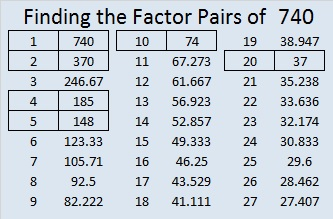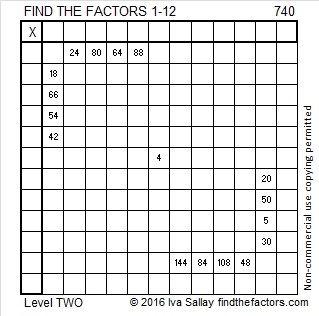# 740 and Level 2

• 740 is a composite number.
• Prime factorization: 740 = 2 x 2 x 5 x 37, which can be written 740 = (2^2) x 5 x 37
• The exponents in the prime factorization are 2, 1, and 1. Adding one to each and multiplying we get (2 + 1)(1 + 1)(1 + 1) = 3 x 2 x 2 = 12. Therefore 740 has exactly 12 factors.
• Factors of 740: 1, 2, 4, 5, 10, 20, 37, 74, 148, 185, 370, 740
• Factor pairs: 740 = 1 x 740, 2 x 370, 4 x 185, 5 x 148, 10 x 74, or 20 x 37
• Taking the factor pair with the largest square number factor, we get √740 = (√4)(√185) = 2√185 ≈ 27.202941.This level 2 puzzle isn’t too difficult to solve:Print the puzzles or type the solution on this excel file: 12 Factors 2016-01-11

————————————-

Here’s more about 740:

740 is the sum of consecutive numbers several ways:

• 146 + 147 + 148 + 149 + 150 = 740; that’s 5 consecutive numbers.
• 89 + 90 + 91 + 92 + 93 + 94 + 95 + 96 = 740; that’s 8 consecutive numbers.
• 2 + 3 + 4 + 5 + 6 + 7 + 8 + 9 + 10 + 11 + 12 + 13 + 14 + 15 + 16 + 17 + 18 + 19 + 20 + 21 + 22 + 23 + 24 + 25 + 26 + 27 + 28 + 29 + 30 + 31 + 32 + 33 + 34 + 35 + 36 + 37 + 38 = 740; that’s 37 consecutive numbers.

Because 5 and 37 are two of its factors, 740 is the hypotenuse of four Pythagorean triples:

• 240-700-740
• 228-704-740
• 416-612-740
• 444-592-740

740 is also a palindrome in two bases, one of which is double the other:

• 252 BASE 18; note that 2(18²) + 5(18) + 2(1) = 740.
• KK BASE 36 (K = 20 base 10); note that 20(36) + 20(1)

————————————-